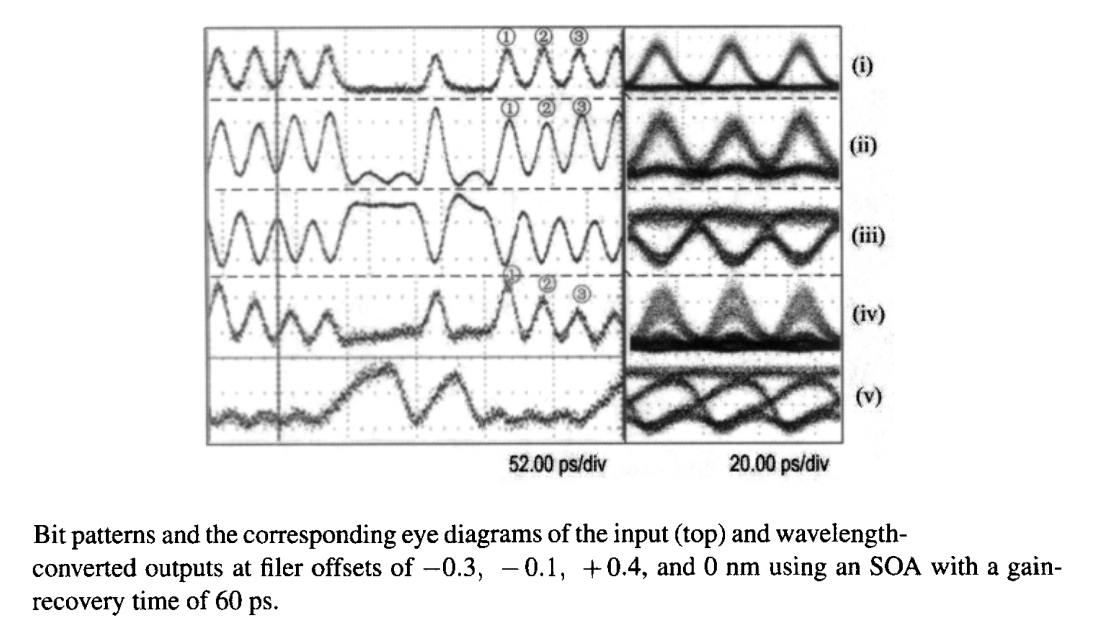Wavelength Converters

Optical networks in which WDM channels are switched based on their carrier wavelengths require a device that can change the carrier wavelength of the channel without affecting its bit pattern that contains the information being transmitted. A large number of such devices have been developed based on both optical fibers and semiconductor materials. This tutorial considers some of them and focuses on their performance from a system standpoint.

1. XPM-Based Wavelength Converters

We first focus on fiber-based wavelength converters in which the nonlinear phenomenon of XPM is employed for optical switching. The basic idea behind this technique has been discussed in the nonlinear techniques and devices tutorial in the context of XPM-induced switching and is illustrated schematically in the figure below.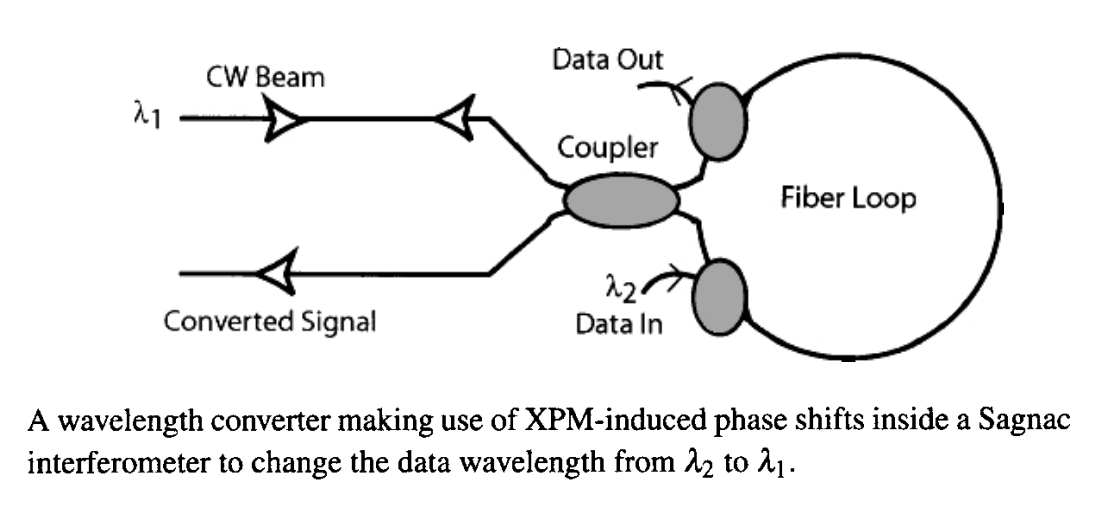The data channel whose wavelength λ2 needs to be changed is propagated inside a fiber of appropriate length together with a CW seed whose wavelength λ1 is chosen to coincide with the desired wavelength of the converted signal. It acts as a pump and imposes an XPM-induced phase shift on the CW seed only in the time slots associated with the 1 bits. This phase shift is converted into amplitude modulation using an interferometer. In practice, a NOLM acting as a Sagnac interferometer is employed for this purpose. The new feature in this figure is that the data channel is launched such that it affects the CW seed in only one direction, resulting in a differential phase shift that can be used to copy the bit pattern of the data channel onto the transmitted λ1 signal.

In a 1994 experiment, the wavelength of a 10-Gb/s data channel was shifted by 8 nm using 4.5-km of dispersion-shifted fiber inside a NOLM. By the year 2000, this technique provided wavelength converted capable of operating at bit rates of up to 40 Gb/s. The NOLM was made using 3 km of dispersion-shifted fiber with its zero-dispersion wavelength at 1555 nm, and it was used to shift the wavelength of a 1547-nm channel by as much as 20 nm. The on-off ratio between the maximum and minimum transmission states was measured to be 25 dB. The optical eye diagrams of the original and wavelength-converted signals indicated that individual pulses were almost unaffected during wavelength conversion.

One may ask what limits the extent of wavelength shift when a NOLM is used for wavelength conversion. The answer is related to different group velocities associated with the two fields interacting through XPM. To estimate the maximum wavelength shift, let us assume that L should be less than the walk-off wavelength LW. Noting that, for two channels separated in frequency by δν, the walk-off parameter dW is related to fiber dispersion as dW = |β2|(2πδν), the condition L < LW reduces to

δν < T0/(2πβ2L)

The required fiber length is obtained from the requirement that φmax = 2γ1P0L must equal π for wavelength conversion to occur. For a dispersion-shifted fiber, L should exceed 1 km if the input peak power P0 is limited to near 1 W. Using L = 2 km and T0 = 20 ps with β2 = 1 ps2/km, the frequency shift δν is close to 1.5 THz, a value that corresponds to a wavelength difference of 12 nm.

The required fiber length can be reduced considerably by employing highly nonlinear fibers for which γ exceeds 10 W-1/km. In a 2001 experiment, wavelength conversion of 0.5-ps pulses was realized using a NOLM with just 50 m of a highly nonlinear fiber with γ = 20.4 W-1/km. Because of such a short length of the fiber loop, the wavelength could be shifted by 26 nm even for such short pulses. The peak power of input pulses required for a π phase shift was close to 4 W in this experiment.

It is not essential to use an optical interferometer for XPM-based wavelength converters. In a simpler approach shown schematically in the figure below, the bit stream acting as the pump is launched into nonlinear fiber together with a CW probe, and the output is passed through a suitable optical filter. Pump pulses at the wavelength λ2 affect the spectrum of the CW probe (at the desired wavelength λ1) through XPM only in the time slots associated with the 1 bits. If the passband of the optical filter is shifted from λ1 by a suitable amount, the output is a replica of the original bit stream at the new wavelength. Any optical filter with a bandwidth larger than that of the data channel (0.5 nm or so) can be used for this purpose, including a fiber grating. In a 2000 experiment, the wavelength of a 40-Gb/s signal was shifted by several nanometers through XPM inside a 10-km-long fiber. This experiment employed a 4-m loop made of polarization-maintaining fiber as a notch filter. The magnitude of wavelength shift was limited by the 10-km length of the fiber in which XPM occurred.

Much larger wavelength shifts have been realized by employing highly nonlinear fibers. In a 2001 experiment, wavelength conversion at a bit rate of 80 Gb/s was performed by using such a 1-km-long fiber with γ = 11 W-1/km. The zero-dispersion wavelength of the fiber was 1552 nm with a relatively small dispersion slope near this wavelength. The 80-Gb/s data channel at the 1560-nm wavelength was first amplified to the 70-mW power level then coupled into the fiber together with a CW probe whose wavelength was varied in the range of 1525-1554 nm. A tunable optial filter with a 1.5-nm bandwidth was used at the fiber output to produce the wavelength-converted channel. Figure (a) below shows the optical spectra just before and after the optical filter at a probe wavelength of 1545.6 nm. Before the filter, the probe spectrum shows multiple sidebands generated through XPM with a dominant peak at 1545.6 nm. After the filter, the carrier has been suppressed relative to the sidebands, resulting in a wavelength-converted signal with a bit stream identical to that of the original channel. The pulse width of the converted signal is shown in figure (b) below as a function of probe wavelength. As seen there, the width remains nearly unchanged over a wide bandwidth. The BER measurements indicated a negligible power penalty for such a wavelength converter.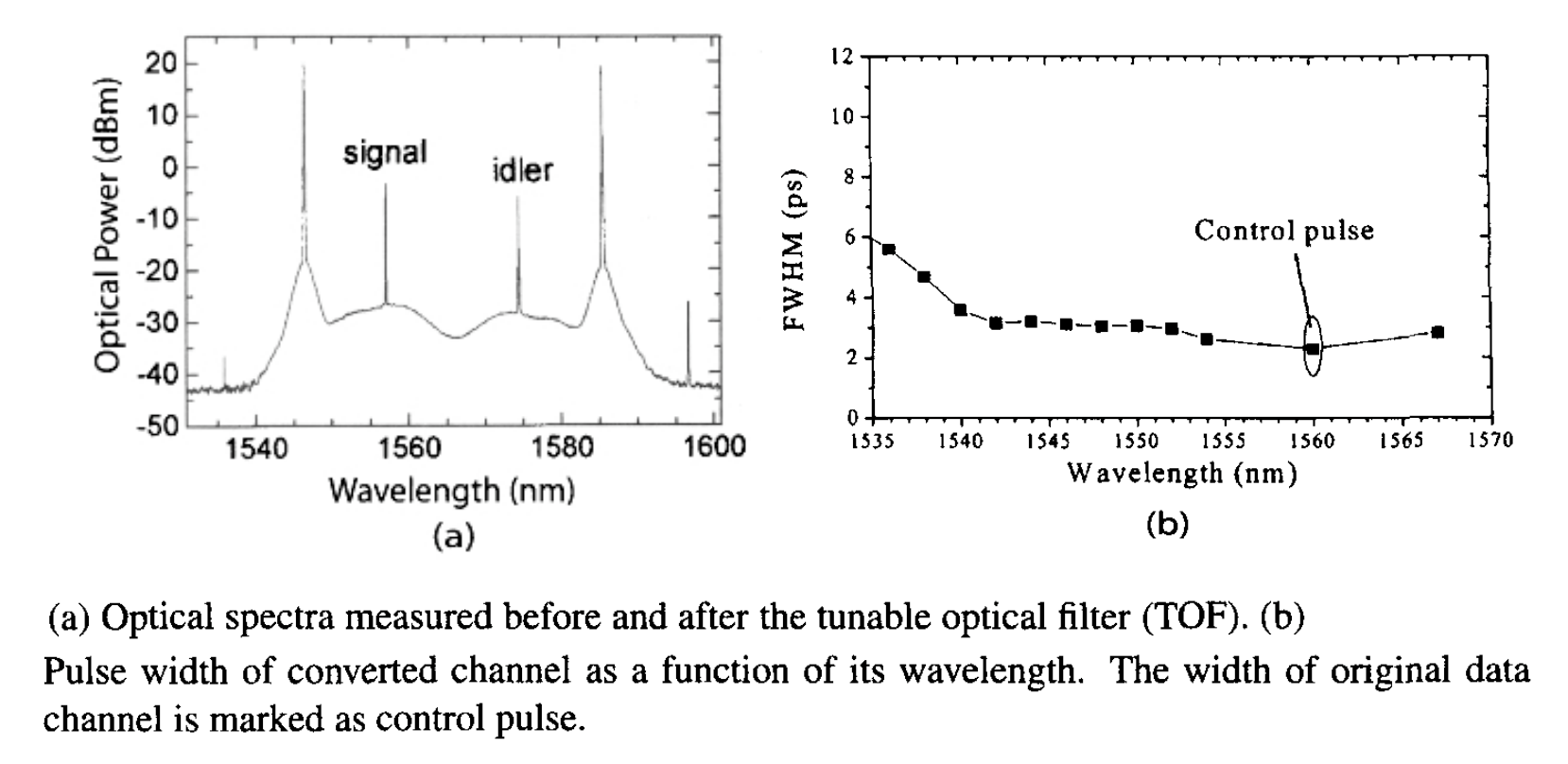Wavelength conversion at a bit rate of 160 Gb/s was realized in a 2004 experiment in which a 0.5-km-long dispersion-shifted fiber was employed to produce the XPM-induced phase shift. This fiber was followed with a fiber grating that acted as a notch filter and two other optical filters with bandwidths of 5 and 4 nm whose pass bands were offset from the carrier frequency of the input signal by 160 GHz. It was observed that the wavelength converter maintained the phase of the input signal, a desirable property from the system standpoint. The performance of such wavelength converts improves further if the Raman gain of the fiber is utilized to enhance the XPM-induced phase shift by pumping the fiber in the backward direction, as indicated in the previous figure. In a 2005 experiment, the efficiency improved by 21 dB when a 1-km-long highly nonlinear fiber was pumped with 600-mW of pump power.

Fiber lengths required to impose sufficient XPM-induced phase shifts can be reduce to below 10 m if microstructured fibers with an ultrasmall effective mode area are employed. Indeed, only 5.8 m of a highly nonlinear fiber was needed in a 2003 experiment in which a fiber grating was used as a narrowband tunable filter. The wavelength of the converted channel was tunable over a 15-nm bandwidth in the normal dispersion region of the fiber. Such a large bandwidth was possible because of a reduction in the walk-off effects for short fibers. The use of normal dispersion eliminates the coherence degradation occurring in the case of anomalous dispersion because of the amplification of laser-intensity noise through modulation instability.

Even shorter fiber lengths are possible if one makes use of a non-silica fiber with a relatively large value of the parameter n2. In a 2006 experiment, only a 1-m-long piece of bismuth-oxide fiber was used to change the wavelength of a 10-Gb/s NRZ signal by as much as 15 nm. The fiber exhibited normal dispersion of 330 ps2/km with γ ≈ 1100 W-1/km at the 1550-nm wavelength. Because of its short length, the onset of SBS was not an issue as the SBS threshold was >1 W. For the same reason, the walk-off effects were negligible. The wavelength conversion in this experiment was based on the XPM-induced nonlinear polarization rotation that makes the fiber act as a Kerr shutter. More specifically, the data channel causing the XPM and the CW beam are launched such that their linear states of polarization are oriented at 45°. The XPM-induced phase shift changes the polarization of the CW beam only in time slots of 1 bits but leaves the polarization of 0 bits unchanged. A polarizer was used to select the wavelength-converted bits.

A practical issue with most wavelength converters is related to the polarization-sensitive nature of XPM in optical fibers. It is well known that the XPM-induced nonlinear phase shift depends on the relative states of polarization of the pump and probe waves, and it acquires its maximum value when the two waves are copolarized. The polarization state of a data channel in a lightwave system is not fixed and can change with time in a virtually random fashion, and such polarization changes would manifest as fluctuations in the peak power of wavelength-converted pulses. It turns out that a polarization-insensitive wavelength converter can be realized by twisting the highly nonlinear fiber in which XPM occurs such that the fiber acquires a constant circular birefringence. In a 2006 experiment, this technique was employed to convert the wavelength of a 160-Gb/s data channel with only 0.7 dB polarization sensitivity. Optical fibers exhibiting circular birefringence are useful for a variety of optical signal-processing applications.

2. FWM-Based Wavelength Converters

FWM-based wavelength converter requires a parametric amplifier such as the one shown in the following figure.If the frequency ωs of a signal needs to be converted to ωi, it is launched inside the fiber together with a CW pump whose frequency ωp is exactly in the middle so that the energy conservation condition, 2ωp = ωs + ωi, is satisfied. As discussed in the nonlinear techniques and devices tutorial, such a FWM process generates an idler wave that mimics the bit pattern of the signal channel precisely because FWM occurs only during temporal slices allocated to 1 bits. No idler photons are produced during 0 bits because both the pump and signal photons must be present simultaneously for FWM to take place. As a result, if an optical filter is placed at the output end of the fiber that passes the idler but blocks the pump and signal, the output is a wavelength-converted replica fo the original bit stream. Although the use of fibers for wavelength conversion was investigated as clearly as 1992, the technique matured only after the advent of highly nonlinear fibers, whose use reduced the length of the fiber in which FWM takes place.

The conversion efficiency, defined as the ratio of output idler power at the end of a fiber of length L to the input signal power, is found to be

ηc = Pi(L)/Ps(0) = (γP0/g)2sinh2(gL)

This equation shows that ηc can exceed 1 when the phase-matching condition is nearly satisfied. In fact, ηc = sinh2(γP0L) ≫ 1 when κ = 0 and γP0L > 1. Thus, FWM-based wavelength converters can amplify a bit stream, while switching its wavelength from ωs to ωi. This is an extremely useful feature of such wavelength converters. Of course, the signal is also amplified. In essence, one has two amplified copies of the input bit stream at two different wavelengths.

It follows from the equation above that the conversion efficiency ηc depends on the phase mismatch κ and the fiber length L through the product κL. It turns out that the range of detuning δ ≡  ωp - ωs over which κL can be made small shrinks rapidly for long fibers. This feature can be seen more clearly from the relation

κ = β2δ2 + 2γP0

where β2 ≈ (ωp - ω03 and β3 is the third-order dispersion parameter at the zero-dispersion frequency ω0 of the fiber. For a given value of δ, the phase mismatch κ can be made zero by choosing the pump wavelength in the anomalous-dispersion regime such that β2 = -2γP02. However, if the signal wavelength deviates from this specific value of δ, ηc decreases at a rate the depends on the fiber length L. As a result, the bandwidth over which wavelength conversion can be realized for a specific pump wavelength is relatively narrow for long fibers (<10 nm for L > 10 km), but it can be increased to beyond 80 nm for fibers shorter than 100 m.

Experimental results on wavelength conversion are in agreement with this simple prediction of FWM theory. In the original 1992 experiment, the use of a 10-km-long dispersion-shifted fiber restricted the wavelength range to 8 nm or so. By 1998, the use of a 720-m long highly nonlinear fiber with a γ value of 10 W-1/km permitted wavelength conversion over 40 nm with only 600 mW of pump powers. The conversion efficiency varied with signal wavelength but was as high as 28 dB, indicating that the wavelength-converted signal was amplified by up to a factor 630 because of FWM-induced parametric amplification. An added advantage of the wide bandwidth is that such a device can be used to convert wavelengths of multiple channels simultaneously. In a 2000 experiment, simultaneous conversion of 26 channels covering wavelengths ranging from 1570 to 1611 nm bandwidth was realized using a 100-m-long section of a highly nonlinear fiber with γ = 13.8 W-1/km. The conversion efficiency was relatively low in this experiment (close to -19 dB) because the launched pump power was limited to 200 mW by the onset of SBS.

The onset of SBS becomes even more serious for wavelength converters that employ longer fiber and want to maintain a high conversion efficiency. The SBS threshold is around 5 mW for long fibers (>10 km) and increases to near 50 mW for fiber lengths of 1 km or so. Since fiber-optic parametric amplifiers require pump power levels approaching 1 W, a suitable technique is needed that raises the threshold of SBS and suppresses it over the amplifier length. A common technique used in practice modulates the pump phase either at several fixed frequencies near 1 GHz, or over a broad frequency range using a pseudorandom pattern at a bit rate as high as 10 Gb/s. This technique suppresses SBS by broadening the pump spectrum but it does not affect the parametric gain much. However, the SNR of both the signal and idler is reduced when dispersive effects within the fiber convert phase modulation into amplitude modulation of the pump. Pump-phase modulation also leads to broadening of the idler spectrum, making it twice as broad as the pump spectrum. Such broadening of the idler is of concern for wavelength converters: it is avoided in practice using dual-pump parametric amplifiers that also have other advantages.

As seen in the nonlinear techniques and devices tutorial, the dual-pump configuration provides nearly uniform gain over a wide bandwidth, while allowing polarization-independent operation of the device. The suppression of idler broadening can be understood by noting that the complex amplitude Ai of the idler field resulting from the FWM process has the form Ai ∝ Ap1Ap2A*s, where Ap1  and Ap2 are the pump amplitudes. Clearly, the phase of the idler would vary with time if the two pumps are modulated either in phase or in a random manner. However, if the two pumps are modulated such that their phases are always equal but opposite in sign, the product Ap1Ap2 will not exhibit any modulation. As a result, even though the idler spectrum will be a mirror image of the signal spectrum, the bandwidth of the two spectra will be identical. A digital approach makes use of binary phase modulation so that the phase of both pumps is modulated in the same direction but takes only two discrete values, namely 0 and π. This approach works because the product Ap1Ap2 does not change under such a modulation scheme. The polarization problem is solved by using two orthogonally polarized pumps.

Figure (a) below shows the optical spectrum recorded at the output when a 1557-nm signal was launched inside a dual-pump wavelength converter. The two pumps had power levels of 118 and 148 mW at wavelengths of 1585.5 and 1546.5 nm, respectively. The power was higher at the shorter wavelength to offset the Raman-induced power transfer to the longer-wavelength pump. FWM occurred inside a 1-km-long highly nonlinear fiber (γ = 18 W-1/km). The zero-dispersion wavelength of the fiber was 1566 nm with a dispersion slope of 0.027 ps/nm2/km at this wavelength. The idler generated through FWM near 1570 nm had the same bit pattern as the signal. Its average power was also comparable to that of the signal, indicating nearly 100% efficiency for such a wavelength converter. In fact, as shown in figure (b) below, high efficiency could be maintained over a bandwidth of 40 nm or so. The efficiency varied somewhat with the signal SOP but variations were below 2 dB over a 30-nm range. The wavelength of multiple channels can be converted simultaneously by such parametric amplifiers. It is also noteworthy that a single signal channel creates multiple idlers at different wavelengths that carry the same information as the signal, resulting in the so-called wavelength multicasting.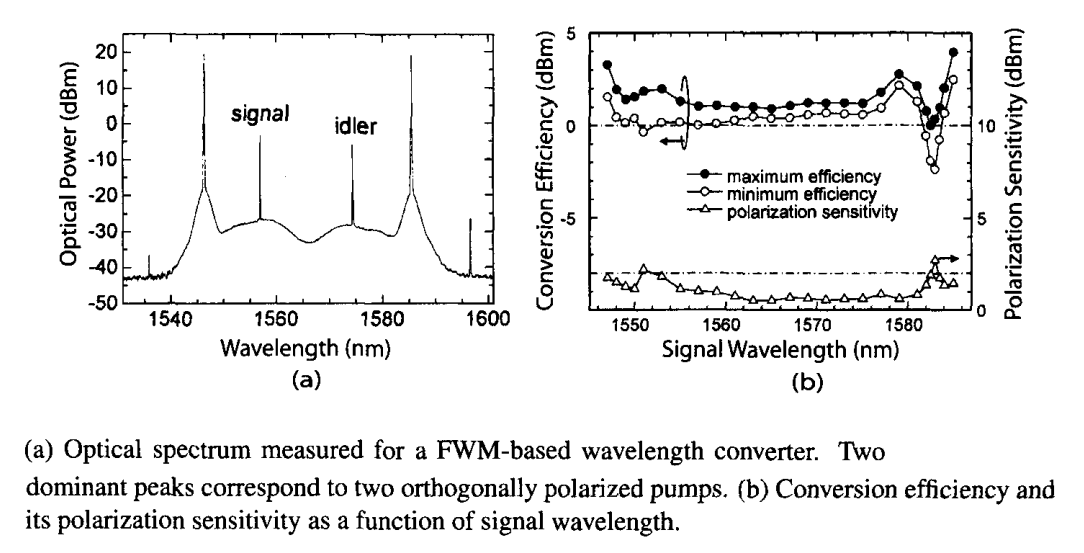Fiber-based wavelength converters have benefited considerably with the advent of photonic crystal fibers (PCFs) and non-silica fibers. A 64-m-long PCF was used in a 2005 experiment. It had a relatively constant dispersion over a 100-nm bandwidth centered near 1550 nm with a small effective mode area. These two features permitted efficient wavelength conversion in spite of a relatively short length of the fiber. In a late experiment, a similar device was used with success for converting the wavelength of a 40-Gb/s signal coded with DPSK format. The highest conversion bandwidth of 100 nm was realized in another 2005 experiment in which the PCF length was reduced to only 20 m.

The shortest fiber length of only 40 cm for a wavelength converter was made possible with the advent of the bismuth-oxide fibers. Such fibers exhibit a blue of the Kerr nonlinearity n2 which is 70 times larger than that of silica fibers. As a result, by reducing the core diameter to below 4 μm, the value of the nonlinear parameter γ can be increased to beyond 1000 W-1/km. Such fibers exhibit FWM even when their length is below 1 m. Moreover, their SBS threshold is large enough that one does not have to modulate the pump phase. In the 2006 experiment, the wavelength of a 40-Gb/s signal could be converted with -16 dB conversion efficiency by launching about 1 W of CW pump power into a 40-cm-long bismuth-oxide fiber. A PCF design was employed in 2007 for a 1-m-long bismuth-oxide fiber to realize wavelength conversion of 10-Gb/s channels over a 35-nm bandwidth.

3. Passive Semiconductor Waveguides

In place of optical fibers, passive semiconductor waveguides can be employed for wavelength conversion. FWM in silicon waveguides has been used for wavelength conversion in several recent experiments. As discussed earlier in the nonlinear techniques and devices tutorial, the performance of such waveguides is limited by free carriers that are generated through TPA when the waveguide is pumped continuously near 1550 nm. For typical values of the carrier lifetime in silicon waveguides (>1 ns), the density of free carriers builds up to the extent that losses induced by them reduce the conversion efficiency to below -10 dB under CW pumping. For this reason, early experiments solved this problem by employing picosecond pump pulses.

CW pumping was employed in a 2006 experiment in which the use of 160-mW of pump power inside a 2.8-cm-long silicon waveguide provided a conversion efficiency of -10.6 dB for a 10-Gb/s channel. The channel bit rate was soon extended to 40 Gb/s in a 2006 experiment in which the effective carrier lifetime was reduced by using a reversed-biased silicon waveguide. A conversion efficiency of -8.6 dB at a pump power of 450 mW could be realized using an 8-cm-long waveguide with 25 V of reverse bias that decreased the density of free carriers by removing them from the region where FWM took place. In another experiment, the objective was to enhance the bandwidth over which wavelength conversion can be realized. By controlling the waveguide dispersion through device dimensions, a bandwidth of more than 150 nm could be realized with pulsed pumping, but the conversion efficiency was limited to below -9.5 dB in this experiment. By 2009, 40-Gb/s wavelength conversion with CW pumping was realized over a bandwidth of more than 50 nm using a 1.1-cm-long silicon waveguide, but the conversion efficiency was only -18 dBm in this experiment. These results show that it will be difficult to realize a net gain at the converted wavelength with silicon waveguides.

LiNbO3 waveguides provide an alternative approach. They exhibit a finite second-order susceptibility χ(2) that can be used for three-wave mixing, a process for which the idler frequency is given by ωi = ωp - ωs when pump and signal waves interact inside the waveguide. This process is also called difference-frequency generation, and it requires a phase-matching condition to be satisfied. As early as 1993, a LiNbO3 waveguide was used for wavelength conversion by employing the technique of periodic poling in which the sign of χ(2) is inverted periodically along the waveguide length for realizing quasi-phase matching.

Such devices require the use of a single-mode pump laser operating in the spectral region near 780 nm with 50-100 mW of power. In practice, it is difficult to simultaneously couple a 780-nm pump and a 1550-nm signal into the fundamental mode of the waveguide. Al alternative scheme makes use of two cascaded second-order nonlinear processes occurring in a periodically poled LiNbO3 (PPLN) waveguide using a pump laser operating near 1550 nm. In such a wavelength converter, the pump at a frequency ωp is first up-converted to 2ωp through second-harmonic generation, which then generates wavelength-shifted output through difference-frequency generation. Such a cascaded process mimics FWM but is much more efficient than FWM based on third-order susceptibility. It is also less noisy than the FWM process occurring inside the active region of SOAs.

Multiple WDM channels can be converted simultaneously using a PPLN waveguide. Figure (a) below shows the optical spectrum recorded in a 1999 experiment in which four channels at wavelengths in the range of 152 to 1558 nm were coupled into a PPLN waveguide together with a 1562-nm pump with 110 mW power. The four peaks on the right side of the pump peak represent the four wavelength-converted channels. The conversion efficiency is about 5% for all channels but it can be increased by increasing pump power. The inset shows the wavelength conversion of a single channel with 16% efficiency at a pump power of 175 mW. The conversion efficiency depends on the wavelength separation between the pump and signal, as shown in figure (b) below. The bandwidth of the flat region over which conversion efficiency is nearly constant is more than 60 nm. With a proper device design, conversion efficiencies of >70% have been realized at a pump power of 150 mW.

LiNbO3 devices can respond at femtosecond time scales and thus easily operate at bit rates of 40 Gb/s or more. conversion of 160-Gb/s RZ signals was realized in a 2007 experiment in which two pumps were used to realize tunable wavelength conversion. In this scheme, one pump at a fixed frequency ω1 first combines with the signal at ωs to generate the sum frequency ω+ = ω1 + ωs. This new wave then combines with the second pump at a tunable frequency ω2 to generate the difference frequency ωi = ω+ - ω2. Such a scheme looks superficially similar to the dual-pump FWM in optical fibers but the idler frequency created by it, ωi = ω1  - ω2 + ωs, is different from that found in the fiber case. Its main advantage is that it provides additional control over the wavelength-conversion process.

4. SOA-Based Wavelength Converters

Several nonlinear techniques for wavelength conversion make use of SOAs. FWM inside an SOA was used for this purpose during the 1990s, and it remains a strong candidate. It is surprising that FWM can occur in SOAs because the carrier density cannot respond at the beat frequency of the pump and signal when the two differ by more than 10 GHz. The nonlinearity responsible for this process has its origin in fast intraband relaxation processes occurring at a time scale of 0.1 ps. As a result, frequency shifts as large as 10 THz, corresponding to wavelength conversion over a range of 80 nm, are possible. For the same reason, this technique can work at bit rates as high as 100 Gb/s and is transparent to both the bit rate and the data format. Because of the gain provided by the amplifier, the conversion efficiency can be quite high, resulting even in a net gain. An added advantage of this technique is the reversal of the frequency chirp since its use inverts the signal spectrum. The performance can be improved further using two SOAs in a tandem configuration.

A simple approach is based on cross-gain saturation, occurring when a weak field is amplified inside the SOA together with a strong field, and the amplification of the weak field is affected by the strong field. To use this phenomenon, the WDM signal whose wavelength λ1 needs to be converted is launched into the SOA together with a low-power CW beam at the wavelength λ2 at which the converted signal is desired. Amplifier gain is mostly saturated by the λ1 beam. As a result, the CW beam is amplified by a large amount during 0 bits (no saturation) but by a much smaller amount during 1 bits. Thus, the bit pattern of the incident signal is transferred to the new wavelength with reverse polarity (1 and 0 bits are interchanged).

The cross-gain saturation technique has been used in many experiments and can work at bit rates as high as 40 Gb/s. It can provide net gain to the wavelength-converted signal. Its main disadvantages are (i) relatively low on-off contrast, (ii) degradation due to spontaneous emission, and (iii) phase distortion because of frequency chirping that invariably occurs in SOAs. The use of an absorbing medium in place of the SOA solves the polarity reversal problem. An electroabsorption modulator has been used for wavelength conversion with success. It works on the principle of cross-absorption saturation. The device blocks the CW signal at λ2 because of high absorption, except when the arrival of 1 bits at λ1 saturates the absorption.

The contrast problem can be solved by using a MZ interferometer, shown schematically in the following figure, with an SOA in its each arm.The CW signal at the wavelength λCW is split equally between eh two arms but the WDM channel at the wavelength λin is sent through only one arm. Durging the 0 bits of this signal, the CW beam is blocked for a suitably balanced interferometer. However, during its 1 bits, the CW beam is directed toward the output port because of a change in the refractive index induced by the WDM signal. The net result is that the output of the MZ interferometer is an exact replica of the incident signal at the wavelength λCW. XPM is the physical mechanism involves in this wavelength-conversion scheme. It is preferable over cross-gain saturation because it does not reverse the bit pattern and results in higher on-off contrast.

Other types of interferometers such as a Sagnac interferometer can also be used with similar results. The MZ interferometer is used in practice because it can be easily integrated on a single chip using InGaAsP/InP technology, resulting in a compact device. The following figure shows a schematic of a widely tunable, wavelength converter with a monolithically integrated tunable semiconductor laser. In this device, the data channel whose wavelength needs to be converted is split into two parts that are fed into two arms of the interferometer with a suitable delay. Such a scheme permits the device to operate at a bit rate of 40 Gb/s in spite of relatively slow gain recovery in two SOAs.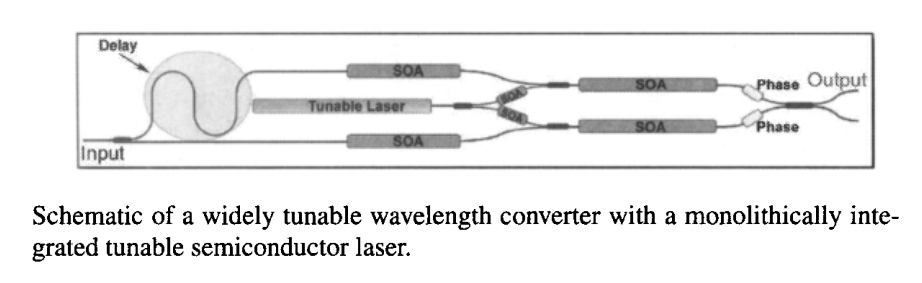A much simpler configuration has been used in several recent experiments in which a single SOA is employed with an optical bandpass filter at its output end whose passband is shifted from the probe wavelength by a small amount. The basic idea is identical to that shown in the first figure except that an SOA replaces the optical fiber as a nonlinear element. In spite of a long gain-recover time of SOAs (~100 ps), such an XPM-based wavelength converter has been shown to work at bit rates as high as 320 Gb/s. The physical process behind it has been termed transient XPM. However, one should keep in mind that cross-gain saturation plays an equally important role in the operation of such a device because it controls the magnitude of XPM-induced spectral broadening. In fact, depending on the exact location of the filter's passband, the wavelength-converted signal may exhibit a bit pattern that is inverted or noninverted with respect to that of the input data. Recall that an inverted bit pattern has its origin in cross-gain saturation. The optical filter plays an important role in pulse shaping. It can even convert an inverted bit stream into a noninverted one.

The following figure shows the results of an experiment in which the wavelength of a 40-Gb/s RZ channel was down-shifted by about 6 nm using an SOA while the filter offset from the wavelength of the CW probe was varied from -0.3 to +0.4 nm. Top row (i) shows the input bit pattern and the corresponding eye diagram. Remaining 4 rows (ii to v) show the wavelength-converted output for a filter offset of -0.3, -0.1, +0.4, and 0 nm, respectively. The bit pattern is not inverted when the offset is -0.3 nm, but it becomes inverted when the offset is reduced to -0.1 nm. The eye diagrams are reasonable in both cases although amplitude jitter is enhanced because of pattern effects. The situation is worse for an offset of +0.4 nm. The worst-case situation (v) occurs for an offset of 0 nm because the effects of cross-gain saturation and slow gain recovery dominate in this case. Similar effects have been seen at higher bit rates but the transient XPM inside SOAs has been successfully used for converting wavelengths of channels operating at 160 and 320 Gb/s.